Note: The other languages of the website are Google-translated. Back to English

## 如何在Excel中将IF函数与AND，OR和NOT一起使用？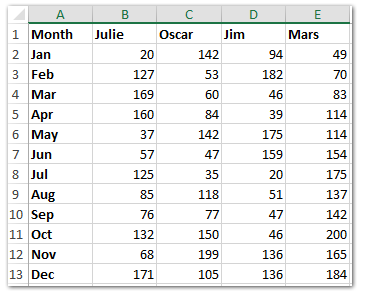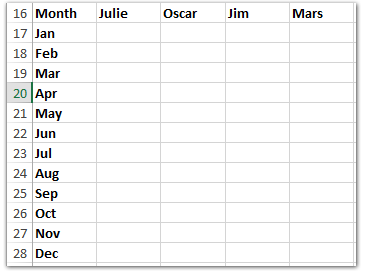#### 如何在Excel中使用IF函数

Excel中IF函数的基本形式如下所示： = IF（逻辑测试，value_if为true，value_if_false）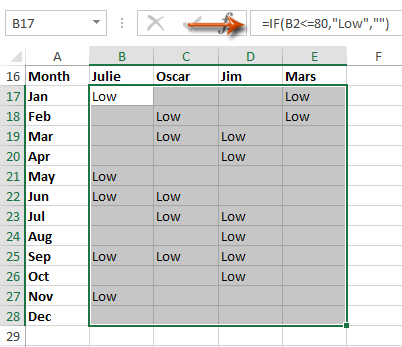#### 轻松地将多个工作表/工作簿/ CSV文件组合到一个工作表/工作簿中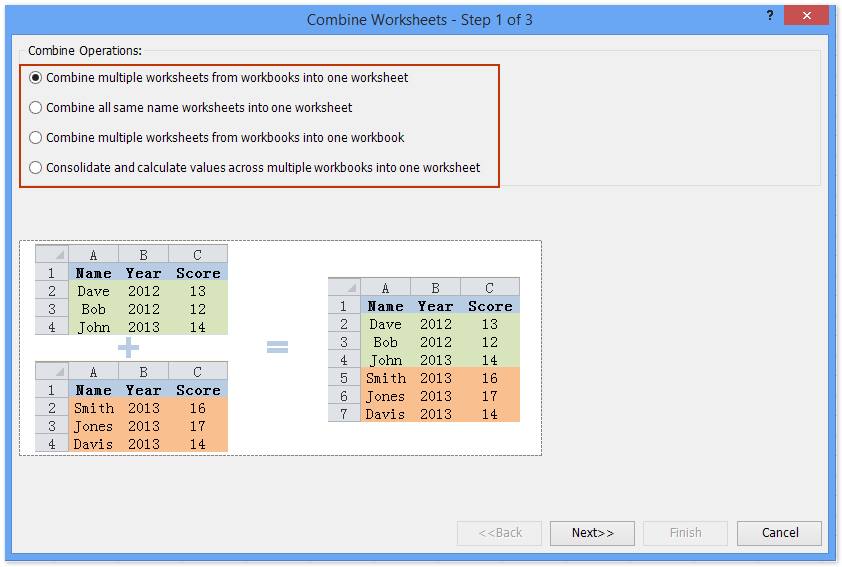#### 如何在Excel中使用If函数和AND函数

Excel中AND函数的基本形式是 = AND（逻辑1，逻辑2，逻辑3等）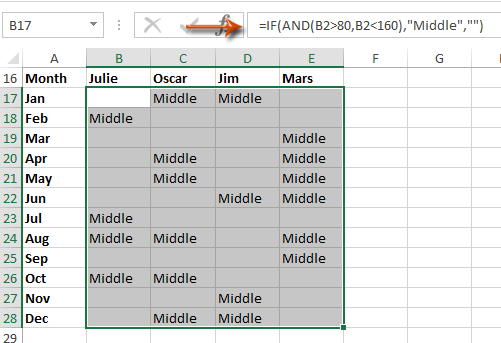#### 如何在Excel中使用IF函数和OR函数

Excel中OR功能的基本形式是 = OR（逻辑1，逻辑2，逻辑3，等等）#### 如何在Excel中将多个IF函数合并为一个公式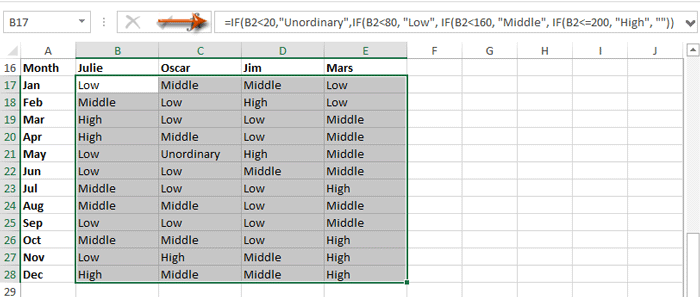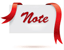公式太难记了吗？ 将公式另存为自动文本条目，以供日后再次使用！ 阅读全文...     免费试用

### 最佳办公效率工具

#### Kutools for Excel解决了您的大多数问题，并使您的生产率提高了80％

• 重用: 快速插入 复杂的公式，图表 以及您以前使用过的任何东西； 加密单元 带密码 创建邮件列表 并发送电子邮件...
• 超级公式栏 （轻松编辑多行文本和公式）； 阅读版式 （轻松读取和编辑大量单元格）； 粘贴到过滤范围...
• 合并单元格/行/列 不会丢失数据； 拆分单元格内容； 合并重复的行/列...防止细胞重复； 比较范围...
• 选择重复或唯一 行; 选择空白行 （所有单元格都是空的）； 超级查找和模糊查找 在许多工作簿中； 随机选择...
• 确切的副本 多个单元格，无需更改公式参考； 自动创建参考 到多张纸； 插入项目符号，复选框等...
• 提取文字，添加文本，按位置删除， 删除空间; 创建和打印分页小计； 在单元格内容和注释之间转换...
• 超级滤镜 （将过滤方案保存并应用于其他工作表）； 高级排序 按月/周/日，频率及更多； 特殊过滤器 用粗体，斜体...
• 结合工作簿和工作表; 根据关键列合并表； 将数据分割成多个工作表; 批量转换xls，xlsx和PDF...
• 超过300种强大功能。 支持Office / Excel 2007-2019和365。支持所有语言。 在您的企业或组织中轻松部署。 完整功能30天免费试用。 60天退款保证。#### Office选项卡为Office带来了选项卡式界面，使您的工作更加轻松

• 在Word，Excel，PowerPoint中启用选项卡式编辑和阅读，发布者，Access，Visio和Project。
• 在同一窗口的新选项卡中而不是在新窗口中打开并创建多个文档。
• 每天将您的工作效率提高50％，并减少数百次鼠标单击！how do i make this formulas talk to one another: =IF(OR(AND(MID(K2,6,1)="N",(MID(K2,6,1)="C"),(MID(K2,6,1)="H"),(MID(K2,6,1)="I"),(MID(K2,6,1)="B"),(MID(K2,6,1)="F"),(MID(K2,6,1)="L"),(MID(K2,6,1)="M"),(MID(K2,6,1)="P"),(MID(K2,6,1)="R"),(MID(K2,6,1)="P"),ISTEXT(G2)="61"),OR(AND(RIGHT(K2,2)=G2))),"Good","Review") =IF(AND(RIGHT(K2,2)=G2),"Good","Review") or =IF(NOT(OR(AND(MID(K2,6,1)="N",(MID(K2,6,1)="C"),(MID(K2,6,1)="H"),(MID(K2,6,1)="I"),(MID(K2,6,1)="B"),(MID(K2,6,1)="F"),(MID(K2,6,1)="L"),(MID(K2,6,1)="M"),(MID(K2,6,1)="P"),(MID(K2,6,1)="R"),(MID(K2,6,1)="P"),ISTEXT(G2)="61"),(RIGHT(K2,2)=G2))),"Good","Review")

",0)))))))

Try this: =IF(OR(H16=0,H16=""),"A",IF(H16=8,"R",IF(H16&lt;7.9,"L","OT")))
Anh Nguyen

=IF(OR((I2=(T2+U2),(I2=(U2+V2)),"Closed","OPEN")

=IF(OR(I2=T2+U2,I2=U2+V2),"CLOSED","OPEN")

=IF(AND(B2="Prudential",OR(C2={"10 年期限","15 年期限","20 年期限","30 年期限"})),D2*0.75,(IF( C2="UL",D2*0.8,"0")))

Anh Nguyen

5 = 100％
4= 99.9% 至 90%
3= 90% 至 85%
2= 85% 至 80%
1= &lt;79.99

OR A1 = "TOPS" AND A9 <5，则为 5，否则为 A9
FG

=ROUND((IFERROR((IF(OR(AND(\$I9="BOTTOMS",BL9&gt;=\$BY\$3),AND(\$I9="TOPS",BL9&gt;=\$BY\$4)),BL9,VLOOKUP(\$I9,\$BX\$3:\$BY\$4,2,FALSE))),0)),0)

I9 - 根据输入的商品代码填充“BOTTOMS”或“TOPS”
BL9 - 商店指数定义的最小集合
BY9 - 买方定义的最小设置（即底部的 14 个单位和顶部的 5 个单位）
BX\$3:\$BY\$4 - 如果 if 语句为假，则查找买方定义的最小集合
FG

KJ

1. 这个数字是超过 1000 英镑还是低于 -1000 英镑

2. Plus 是下一个单元格超过 55% 或更多

G

=IF(AND(D26="",E26=""),(C26-L7),IF(AND(D26&lt;&gt;"",E26=""),D26-L7,E26-L7))

III
II
II
II

II
II
II
II

C。 如果工作人员不符合这两个标准，该函数应返回文本 No。

https://support.office.com/en-us/article/IF-function-69aed7c9-4e8a-4755-a9bc-aa8bbff73be2

A 列有数值。 C & D 列有文字。

A1:A2 = 1

A3:A4 = 2

C1=狗
C2=猫
C3=狗
C4：马

D1=猫
D2=狗
D3=马
D4=狗

https://support.office.com/en-us/article/IF-function-69aed7c9-4e8a-4755-a9bc-aa8bbff73be2

=IF(A3&gt;0,"Welcome",IF(B3&gt;0,"Win",IF(C3&gt;0,"Will","HI")))

kelly001

0  产品特性